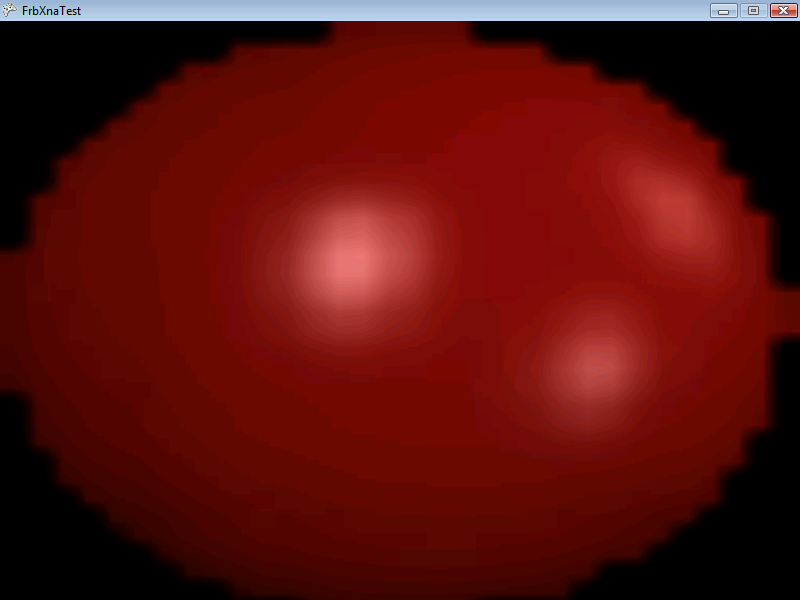# PixelsPerUnitAt

## Introduction

The PixelsPerUnit method is a method that can be used to convert between world units and screen pixels. The PixesPerUnit method can be used if pixel coordinates are desired when the 3D camera is being used.

## Method Signature

```public float PixelsPerUnitAt(float absoluteZ)
public float PixelsPerUnitAt(ref Vector3 absolutePosition)
public float PixelsPerUnitAt(ref Vector3 absolutePosition, float fieldOfView, bool orthogonal, float orthogonalHeight)
```

## Common usage

The most common usage of PixelsPerUnit is to convert from pixels to units. In other words, the most common usage is to obtain “world units per pixel”. Once the number of world units is obtained per pixel, multiplying that value can be multiplied by the desired number of pixels.

To convert “pixels per unit” to “units per pixels”, we simply need to take the reciprocal. If you’re not familiar with this math term, taking the reciprocal of a value is the same as dividing one by the value. The code for this is as follows:

```float pixelsPerUnit = Camera.Main.PixelsPerUnitAt(0);
float unitsPerPixel = 1 / pixelsPerUnit;
int desiredPixels = 64;
float worldUnits = unitsPerPixel * desiredPixels;
```

## Code Example – Setting Sprite Width and Height

The following code example creates a Sprite and scales it to the size of the entire screen. The default resolution is 800 X 600, so scaling the Sprite to this size will make the Sprite fill up the entire screen.

Add the following to Initialize after initializing FlatRedBall:

```// Our default resolution is 800 X 600
int desiredSpritePixelWidth = 800;
int desiredSpritePixelHeight = 600;

// remember, Scale is half of width
sprite.Width= PixelsToUnits(
desiredSpritePixelWidth,
sprite.Z);
sprite.Height = PixelsToUnits(
desiredSpritePixelHeight,
sprite.Z);
```

Add the PixelsToUnits method at class scope:

```float PixelsToUnits(int numberOfPixels, float absoluteZ)
{
return numberOfPixels /
Camera.Main.PixelsPerUnitAt(absoluteZ);
}
```## Code Example – Spacing Objects on Screen

This example creates a row of circles, each touching end-to-end, 32 pixels apart on a 3D camera. It uses PixelsPerUnitAt to size and space the circles. The following code can be placed in a screen’s CustomInitialize  method:

```void CustomInitialize()
{
// This could also be set in Glue by unchecking Is 2D
Camera.Main.Orthogonal = false;

float leftEdge = Camera.Main.AbsoluteLeftXEdgeAt(0);
float unitsPerPixel = 1 / Camera.Main.PixelsPerUnitAt(0);

float firstCircleX = leftEdge + unitsPerPixel * 16;
float circleRadius = unitsPerPixel * 16;
float circleSpacing = unitsPerPixel * 32;

float currentX = firstCircleX;

for(int i = 0; i < 24; i++)
{
Circle circle = ShapeManager.AddCircle();
circle.X = currentX;
currentX += circleSpacing;
}
}
```## PixelsPerUnitAt and FieldOfView

If you are using a 3D Camera, then the FieldOfView impacts the PixelsPerUnitAt method. PixelsPerUnitAt measures the number of pixels per world unit. In other words, the formula is:

PixelsPerUnit = NumberOfPixels / NumberOfWorldUnits

Therefore, increasing NumberOfWorldUnits will decrease the PixelsPerUnitAt. Increasing the FieldOfView will increase the number of visible world units, but will keep the NumberOfPixels constant. Therefore, a larger FieldOfView will decrease PixelsPerUnitAt

## PixelsPerUnitAt and resolution

The resolution of the game impacts the PixelsPerUnitAt value. Increasing the resolution increases the NumberOfPixels in the formula above. Therefore, if your game runs at higher resolution, then the PixelsPerUnitAt will be larger.

Keep in mind that by default the FlatRedBall Camera uses the same FieldOfView. This means that if your game increases its resolution, the PixelsPerUnitAt will increase. To be more specific, the PixelsPerUnitAt uses the resolution height. Therefore, changing the width of the application will not impact the PixelsPerUnitAt, but changing the height will.